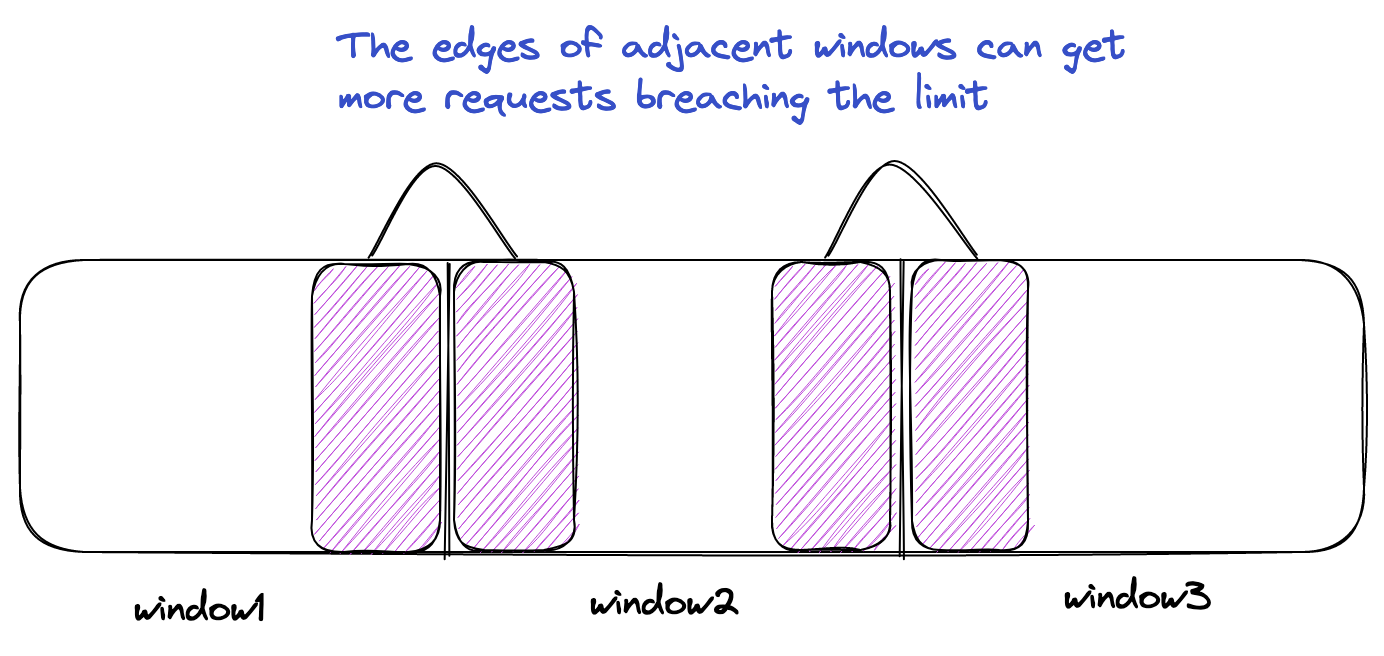# Rate Limiting - Different Approaches and Implementation

## Introduction

In software engineering, rate limiting has so many use cases. A few examples are

1. Limiting the number of times OTP is sent to mobile numbers.
2. Protecting the systems from DDOS (distributed denial of service) attacks.
3. Enterprise SaaS has pricing based on the number of requests per minute - so it is directly related to the business.

In this blog post, we will discuss different approaches of rate limiting.

## First Principle Approach

Let’s start with very basic first-principle thinking. What is rate limiting? let’s break it down. Rate is the velocity at which an object is moving. We measure the velocity of the car in km per hour. Similarly, in computer science, the rate at which the requests are entered into a system is measured in requests per second. Limiting is self-explanatory. So, the problem statement is to control the rate at which the requests are coming to a system.

To measure the rate, we need to store the data somewhere. What are the options we have? hard disk and main memory. The disks are slow — This is not always true! it depends on how we use it! sequential reads and writes are relatively faster in the disk and we can further optimize it with the operating system’s page caching into main memory — so the only option we have is main memory.

There are two popular libraries that store data in the main memory with high-level abstractions. They are Memcached and Redis. We need to leverage them to implement rate limiting. Let’s see how we can do that.

## Token Bucket Algorithm

The idea is simple, we will store the rate limit “X” at a cache key with an expiry of “T” seconds. The cache key can be an API key or a combination of user id, visitor cookie, IP address, etc… with the current minute as a suffix. For example, “u_lokesh1729:04” where the “u_lokesh1729” is the username of the user and “04” is the current minute.

1. Get the rate limit of a given cache key.

1. set the key with “T” seconds as TTL
2. set the value as “X”
3. If the key is found

1. If the limit has been breached, reject the request.
2. If not, allow the request
``````import os
import redis

RATE_LIMIT = os.env.get("RATE_LIMIT", 50)

def rate_limit(key, rate):
res = redis.get(key)
if res is not None and res > RATE_LIMIT:
raise TooManyRequestsException("rate limit breached")
redis.incr(key)
redis.expiry(key, 60)``````

## Modified Token Bucket Algorithm

There is a slightly different approach for the same algorithm. Instead of adding the current minute to the key, we store two cache keys. One is mapping between the API key and the number of tokens. Another is a mapping between the API key and the last filled timestamp of the bucket in the epoch.

An example would be, “u_lokesh1729 -> 100” and “u_lokesh1729 -> 1674378362”.

How do we determine the breach?

1. Get the count and the created timestamp.

2. If the count is greater than the limit, reject the request.

3. Else, find the diff between the current timestamp and the last filled timestamp of the bucket.

4. If the diff is greater than “T” seconds meaning the last window had already crossed.

1. Reset the rate limit and timestamp. There is no need to set the expiry. These are persistent keys.
5. Else

1. Compute and set the remaining tokens. How to compute? Say, the rate limit is “x” req/minute, and the diff between the bucket timestamp and the current timestamp is “y” seconds. The remaining tokens would be `(y/1000) * x`

## Sliding Window Approach

### Burst at edges

The downside of the above approach is that it is missing an edge case. The corners of adjacent windows can breach the rate limit but the algorithm won’t be able to identify that. Refer to the below picture.### No Atomicity

Redis provides `MULTI` the command which wraps multiple redis commands and executes them as a single atomic block. So, all of them succeed or fail not partially. But, if we look at the above approaches, they cannot be fit in an atomic block because there is a business logic (checking the timestamp, tokens, etc…) happening outside. It can be solved with LUA scripting but that would complicate things.

### Redis sorted sets

Redis provides a data structure called sorted sets. It is nothing but an array of tuples sorted by the score. An example would be

``````{
"key": [
(10, "abcd"),
(11, "def"),
(15, "xyz")
]
}``````

The `key` is the redis key at which the sorted set is stored. The TTL is set at the key level. The first item in the tuple is the score by which the list is stored. The second item in the tuple is the member associated with the score.

So, how do we use sorted sets to implement rate limiting? simple, we will use the epoch value of the timestamp as the score.

1. Remove the older entries which pass the current time using `ZREMRANGEBYSCORE key -inf <curr_timestamp - 1 minute>` .
2. Add the current timestamp to the set using `ZADD key <timestamp> <timestamp>` . We use the timestamp both as the score and the member.
3. Set the expiry of the key to one minute (or T seconds) using `EXPIRE key 60`
4. Get the count of the current values in the sorted set using `ZCARD key`

Using the count we got above, we can determine whether to allow or reject the request. Also, all the above commands are to be run in a single `MULTI` command.

What if the application is using Memcached? we can implement the above approach in the application code like this.

``````import cache
import time

def rate_limit(key, ttl, rate_limit):
items = cache.get(key, [])
time = int(time.time())
# delete the items occurred one interval ago
while items and items[-1] < time:
items.pop()